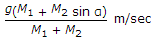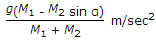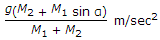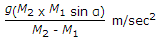# Civil Engineering - Applied Mechanics

### Exercise :: Applied Mechanics - Section 2

21.

When a body of mass M1 is hanging freely and an other of mass M2 lying on a smooth inclined plane(α) are connected by a light indextensible string passing over a smooth pulley, the acceleration of the body of mass M1, will be given by

 A.B.C.D.Explanation:

No answer description available for this question. Let us discuss.

22.

According to Law of Triangle of Forces

 A. three forces acting at a point, can be rep-resented by the sides of a triangle, each side being in proportion to the force B. three forces acting along the sides of a triangle are always in equilibrium C. if three forces acting on a, point can be represented.in magnitude and direction, by the sides of a triangle taken in order, these will be in equilibrium D. if three forces acting at a point are in equilibrium each force is proportional to the sine of the angle between the other two E. if the forces acting on a particle be represented in magnitude and direction by the two sides of a triangle taken in order, their resultant will be represented in magnitude and direction by the third side of the triangle, taken in opposite order.

Explanation:

No answer description available for this question. Let us discuss.

23.

A Second's pendulum gains 2 minutes a day. To make it to keep correct time its length

 A. must be decreased B. must be increased C. is not changed but weight of the bob is increased D. is not changed but weight of the bob is decreased E. none of these.

Explanation:

No answer description available for this question. Let us discuss.

24.

The following is not a law of static friction :

 A. The force of friction always acts in a direction opposite to that in which the body tends to move B. The force of friction is dependent upon the area of contact C. The force of friction depends upon the roughness of the surface D. The magnitude of the limiting friction bears a constant ratio to the normal reaction between two surfaces.

Explanation:

No answer description available for this question. Let us discuss.

25.

The velocity of a moving body, is

 A. a vector quantity B. a scalar quantity C. a scalar as well as a vector quantity D. none of these.